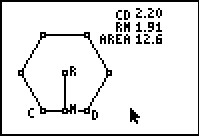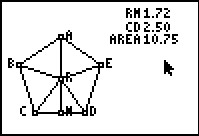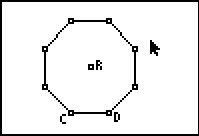••• ##### Device
• TI-83 Plus Family
• TI-84 Plus
• TI-84 Plus Silver Edition
•TI-84 Plus C Silver Edition
•TI-84 Plus CE
• ##### Software

TI Connect™
TI Connect™ CE

• ##### Report an Issue

Geometry: From the Center of the Polygon
by Texas Instruments#### Overview

Students explore the area of a regular polygon in terms of the apothem and the perimeter in order to derive the formula for a regular pentagon and regular hexagon.

#### Key Steps

•Students will investigate the formula for finding the area of a regular polygon given the length of the apothem and the perimeter. First, students will look at a regular pentagon.

•Next, students will investigate a regular hexagon and derive the formula for the area of a regular hexagon.

•Then, in Problem 3, students will apply what they learned in the previous problems to discover the area of a regular polygon in general using an octagon and the triangles created by the radii of the octagon. Finally, students will apply what they have learned to answer questions about the area of regular polygons using the formula they derived.